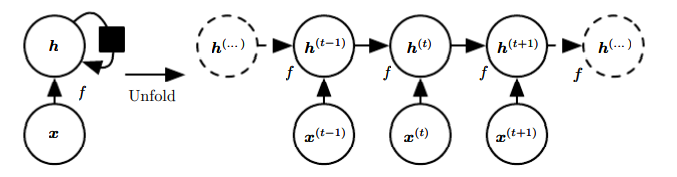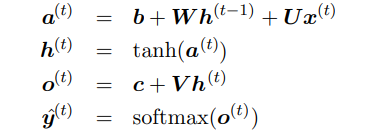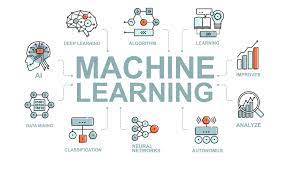Ashray Saini — Published On July 20, 2021 and Last Modified On July 20th, 2021

This article was published as a part of the Data Science Blogathon

## Table Of Content

• Introduction
• Architecture Of Recurrent Neural Network
• Application Of Recurrent Neural Network

## Introduction

Recurrent Neural Networks (RNN) are a part of the neural network’s family used for processing sequential data. For example, consider the following equation:

ht = f(ht-1; x)                                                                e.q 1Figure 1: A recurrent neural network with no output which represents the equation 1. This network takes x as input and incorporates it into state h which is also known as a hidden state that is passed forward. The black square indicates a delay in a single time step.

The above equation is recurrent because the definition of h at time t refers to the same definition at time t-1. If we want to find the value of h at 3rd time step, we have to unfold equation1 i.e.

h3 = f( h2; x) = f(f( h1; x); x)                                              e.q 2

Now the question that arises here is that we already have Feedforward Neural Network(ANN), then why should we use a Recurrent Neural Network. Let’s understand this with an example:

Consider the two sentences
“I went to India in 2017” and “In 2017, I went to India”.

Now, if we ask the model to extract the information on where did the person was in 2017, we would like it to recognize the year 2017, whether it appears in the second or the sixth position of the sentence.

Suppose we give these two sentences to the Feedforward Neural Network, as we know that it has different learning weights for each layer the model will try to learn all of the rules of languages separately at each position in the sentence even though the meaning of both sentences is same it will treat them differently. It can become a problem when there are many such sentences
with the same logical meaning and will always negatively affect the model accuracy.

NOTE: Recurrent Neural Network shares the same learning weight across each time step which is an important property of RNN and thus did not suffer from the above problem.

## Architecture Of Recurrent Neural Network

Figure 2: Architecture of recurrent neural network where x, h, o, L, y represents input, hidden state, output, loss, and target value respectively.

Recurrent Neural Network maps an input sequence x values to a corresponding sequence of output o values. A loss L measure the difference between the actual output y and the predicted output o. The RNN has also input to hidden connection parametrized by a weight matrix U, hidden to hidden connections parametrized by a weight matrix W, and hidden-to-output connections parametrized by a weight matrix V. Then from time step t = 1 to t = n we apply the following equation:Figure 3: These are the forward propagation equations of the recurrent neural network where U, V, W are the weight matrix that is shared among each time step. The above equations are also known as forwarding propagation of RNN where the b and c are the bias vectors and tanh and softmax are the activation functions. To update the weight matrix U, V, W we calculate the gradient of the loss function for each weight matrix i.e. ∂L/∂U, ∂L/∂V, ∂L/∂W, and update each weight matrix with the help of a back-propagation algorithm. When a back-propagation algorithm is applied to RNN, it is sometimes also known as BPTT i.e. backpropagation through time. Gradient calculation requires a forward propagation and backward propagation of the network which implies that the runtime of both propagations is O(n) i.e. the length of the input. The Runtime of the algorithm cannot reduce further because the design of the network is inherently sequential. Depending on the objective we can choose any loss function. Total loss for a given sequence of x values is the sum of all the losses at an individual time step. Another variation that can be done in recurrent neural network architecture is that we can change the recurrent connection from hidden to hidden state and make it from output to hidden state.

NOTE : Such types of recurrent neural networks are less powerful and can express a smaller set of functions this is because of the connection that we have made. Recurrent neural networks which are represented by Figure 2 are universal in the sense that any function computable by a Turing machine can be computed by such a recurrent network of finite size.

## Application of Recurrent Neural Network

RNNs are used in a wide range of problems :

### Text Summarization

Text summarization is a process of creating a subset that represents the most important and relevant information of the original content. For example, text summarization can be useful for someone who wants to read the summary instead of the whole content. It will save time if the original content was not useful for the reader.

### Language Translation

Almost every language translation machine uses RNN in its backend. They are used to convert text from one language to other. Input will be the source language and output will be the language that users want. The most popular example of language translation is Google Translator.

### Language Modelling And Generating Text

Language modelling is the task of assigning a probability to sentences in a language. Besides assigning a probability to every sequence of words, the language models also assign a probability for the likelihood of a given word (or a sequence of words) to follow a sequence of words. For example, nowadays every messenger provides such a facility that tries to autocomplete a sentence and show suggestions while we are typing.

### Chatbots

A chatbot is a computer program that simulates and processes human conversation. Chatbots are often simple as rudimentary programs that answer an easy query with a single-line response or as complex as digital assistants that learn and evolve from their surroundings and gather and process information. For example, most online customer services have a chatbot that responds to queries in a question-answer format.

### Generating Image Descriptions

A Combination of
Convolutional Neural Network and Recurrent Neural Network can be used to create
a model that generates natural language descriptions of images and their
regions. The model will describe what exactly is happening inside an image.

## End Notes:

The source of the images has been taken from Deep Learning by Lan Goodfellow, Yoshua Bengio, and Aaron Courville.

I hope you enjoyed reading the article. If you found it useful, please share it among your friends and on social media. For any queries, suggestions, or any other discussion, please ping me here in the comments or contact me via Email or LinkedIn.